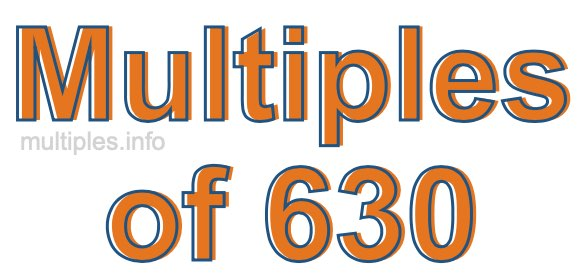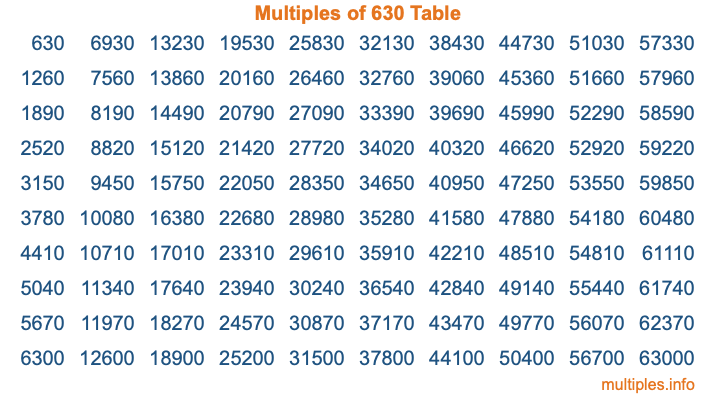Multiples of 630Welcome to the Multiples of 630 page. Here we will first teach you everything you will ever need to know about the multiples of 630, and then give you a study guide summary of everything we taught you to make sure you remember it all. Use this page to look up facts and learn information about the multiples of 630. This page will make you a multiples of six hundred thirty expert!

Definition of Multiples of 630
Multiples of 630 are all the numbers that when divided by 630 equal an integer. Each of the multiples of 630 are called a multiple. A multiple of 630 is created by multiplying 630 by an integer.

Therefore, to create a list of multiples of 630, you start with 1 multiplied by 630, then 2 multiplied by 630, then 3 multiplied by 630, and so on for as long as you want. Thus, the list of the first five multiples of 630 is 630, 1260, 1890, 2520, and 3150. To see a larger list of multiples of 630, see the printable image of Multiples of 630 further down on this page. We also have a category where you can choose any nth multiple of 630.

Multiples of 630 Checker
The Multiples of 630 Checker below checks to see if any number of your choice is a multiple of 630. In other words, it checks to see if there is any number (integer) that when multiplied by 630 will equal your number. To do that, we divide your number by 630. If the the quotient is an integer, then your number is a multiple of 630.

Is  a multiple of 630?

Least Common Multiple of 630 and ...
A Least Common Multiple (LCM) is the lowest multiple that two or more numbers have in common. This is also called the smallest common multiple or lowest common multiple and is useful to know when you are adding our subtracting fractions. Enter one or more numbers below (630 is already entered) to find the LCM.

Check out our LCM Calculator if you need more details about the Least Common Multiple or if you need the LCM for different numbers for adding and subtraction fractions.

nth Multiple of 630
As we stated above, 630 is the first multiple of 630, 1260 is the second multiple of 630, 1890 is the third multiple of 630, and so on. Enter a number below to find the nth multiple of 630.

th multiple of 630

Multiples of 630 vs Factors of 630
630 is a multiple of 630 and a factor of 630, but that is where the similarities end. All postive multiples of 630 are 630 or greater than 630. All positive factors of 630 are 630 or less than 630.

Below is the beginning list of multiples of 630 and the factors of 630 so you can compare:

Multiples of 630: 630, 1260, 1890, 2520, 3150, etc.

Factors of 630: 1, 2, 3, 5, 6, 7, 9, 10, 14, 15, 18, 21, 30, 35, 42, 45, 63, 70, 90, 105, 126, 210, 315, 630

As you can see, the multiples of 630 are all the numbers that you can divide by 630 to get a whole number. The factors of 630, on the other hand, are all the whole numbers that you can multiply by another whole number to get 630.

It's also interesting to note that if a number (x) is a factor of 630, then 630 will also be a multiple of that number (x).

Multiples of 630 vs Divisors of 630
The divisors of 630 are all the integers that 630 can be divided by evenly. Below is a list of the divisors of 630.

Divisors of 630: 1, 2, 3, 5, 6, 7, 9, 10, 14, 15, 18, 21, 30, 35, 42, 45, 63, 70, 90, 105, 126, 210, 315, 630

The interesting thing to note here is that if you take any multiple of 630 and divide it by a divisor of 630, you will see that the quotient is an integer.

Multiples of 630 Table
Below is an image of the first 100 multiples of 630 in a table. The table is in chronological order, column by column. The first column has the first ten multiples of 630, the second column has the next ten multiples of 630, and so on.The Multiples of 630 Table is also referred to as the 630 Times Table or Times Table of 630. You are welcome to print out our table for your studies.

Negative Multiples of 630
Although not often discussed or needed in math, it is worth mentioning that you can make a list of negative multiples of 630 by multiplying 630 by -1, then by -2, then by -3, and so on, to get the following list of negative multiples of 630:

-630, -1260, -1890, -2520, -3150, etc.

Multiples of 630 Summary
Below is a summary of important Multiples of 630 facts that we have discussed on this page. To retain the knowledge on this page, we recommend that you read through the summary and explain to yourself or a study partner why they hold true.

There are an infinite number of multiples of 630.

A multiple of 630 divided by 630 will equal a whole number.

630 divided by a factor of 630 equals a divisor of 630.

The nth multiple of 630 is n times 630.

The largest factor of 630 is equal to the first positive multiple of 630.

630 is a multiple of every factor of 630.

630 is a multiple of 630.

A multiple of 630 divided by a divisor of 630 equals an integer.

630 divided by a divisor of 630 equals a factor of 630.

Any integer times 630 will equal a multiple of 630.

Multiples of a Number
Here you can get the multiples of another number, all with the same attention to detail as we did for multiples of 630 on this page.

Multiples of
Multiples of 631
Did you find our page about multiples of six hundred thirty educational? Do you want more knowledge? Check out the multiples of the next number on our list!